Anúncio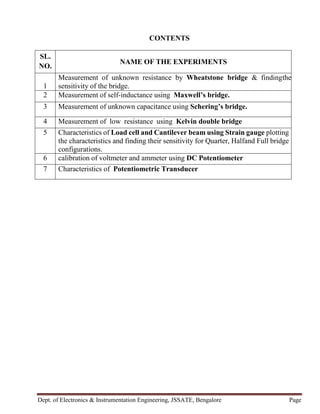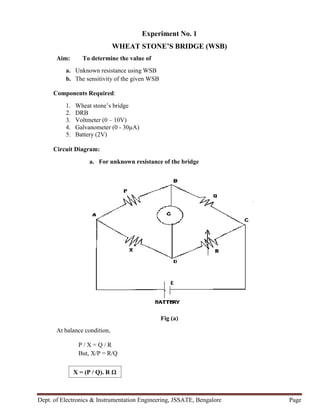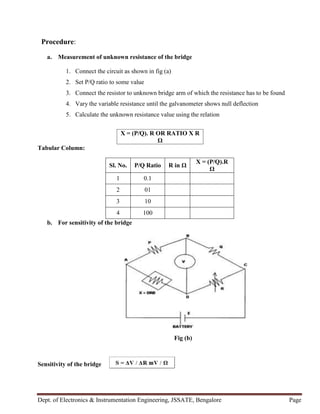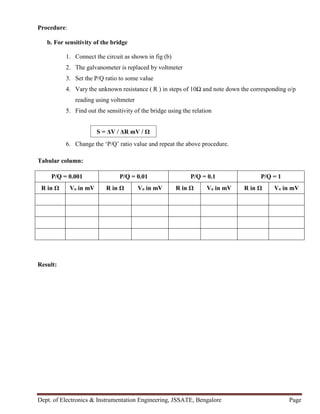Anúncio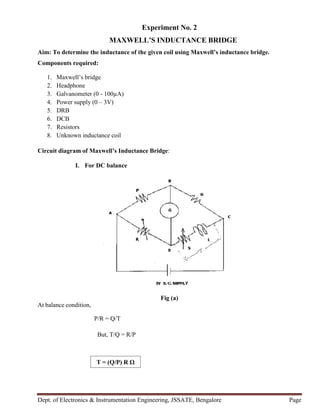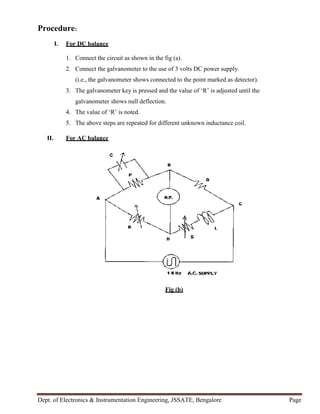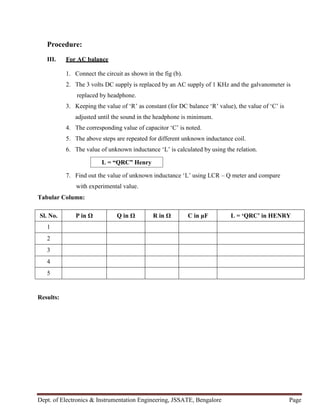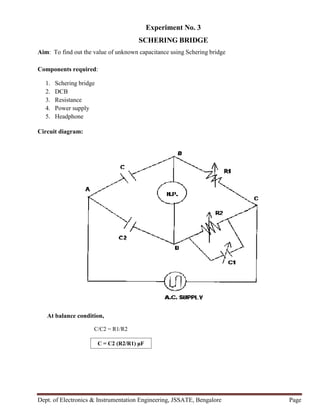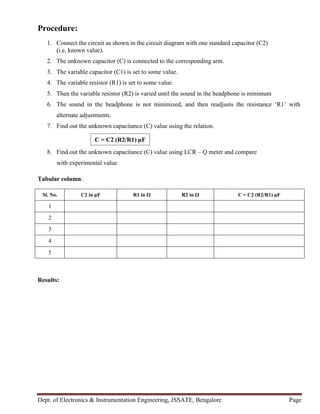Anúncio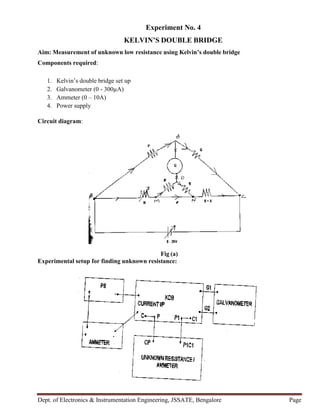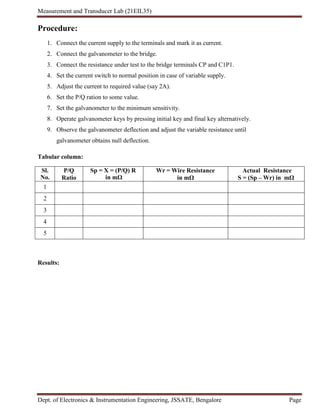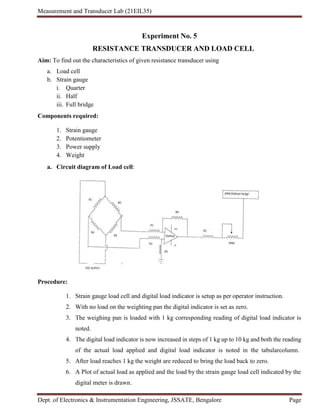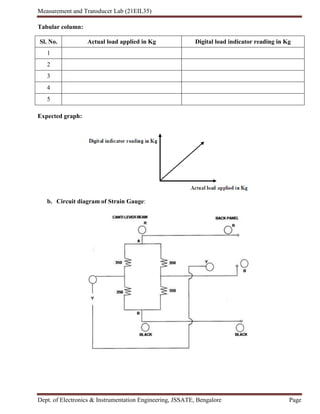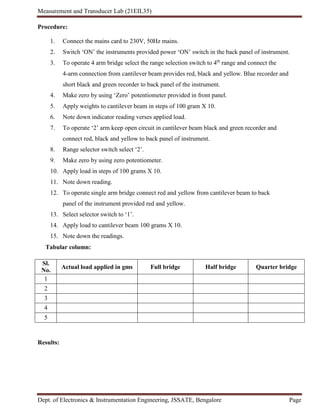Anúncio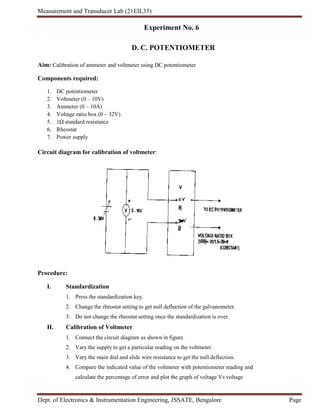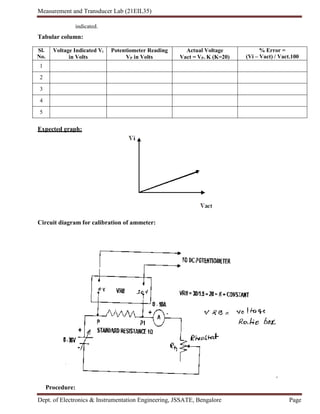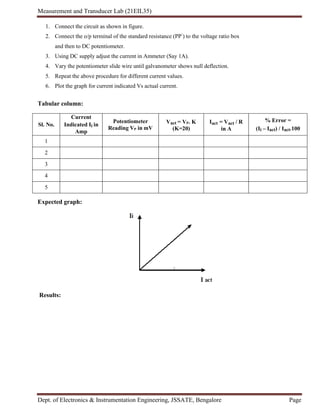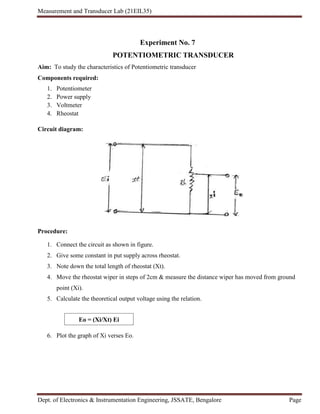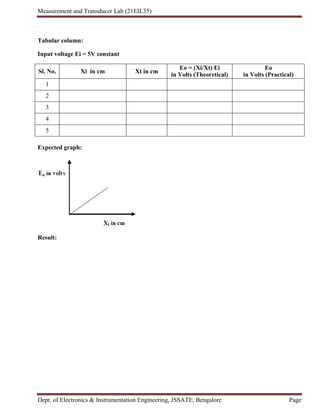Próximos SlideShares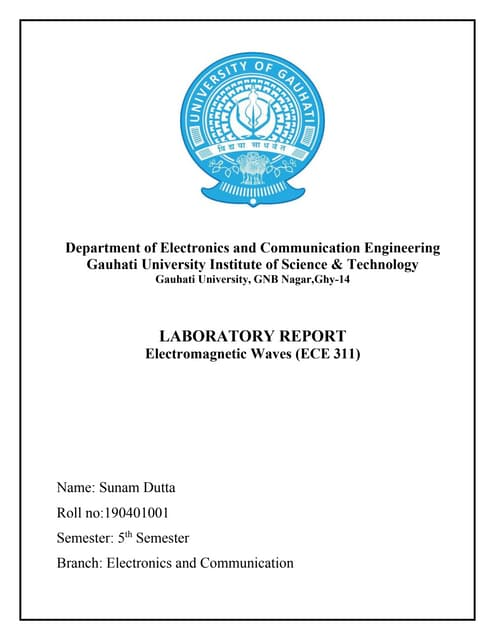Em waves lab assignment converted
Carregando em ... 3
1 de 19
Anúncio

### 2nd cycle experiments.docx

1. Dept. of Electronics & Instrumentation Engineering, JSSATE, Bengalore Page CONTENTS SL. NO. NAME OF THE EXPERIMENTS 1 Measurement of unknown resistance by Wheatstone bridge & findingthe sensitivity of the bridge. 2 Measurement of self-inductance using Maxwell’s bridge. 3 Measurement of unknown capacitance using Schering’s bridge. 4 Measurement of low resistance using Kelvin double bridge 5 Characteristics of Load cell and Cantilever beam using Strain gauge plotting the characteristics and finding their sensitivity for Quarter, Halfand Full bridge configurations. 6 calibration of voltmeter and ammeter using DC Potentiometer 7 Characteristics of Potentiometric Transducer
2. Dept. of Electronics & Instrumentation Engineering, JSSATE, Bengalore Page Experiment No. 1 WHEAT STONE’S BRIDGE (WSB) Aim: To determine the value of a. Unknown resistance using WSB b. The sensitivity of the given WSB Components Required: 1. Wheat stone’s bridge 2. DRB 3. Voltmeter (0 – 10V) 4. Galvanometer (0 - 30µA) 5. Battery (2V) Circuit Diagram: a. For unknown resistance of the bridge Fig (a) At balance condition, P / X = Q / R But, X/P = R/Q X = (P / Q). R Ω
3. Dept. of Electronics & Instrumentation Engineering, JSSATE, Bengalore Page Procedure: a. Measurement of unknown resistance of the bridge 1. Connect the circuit as shown in fig (a) 2. Set P/Q ratio to some value 3. Connect the resistor to unknown bridge arm of which the resistance has to be found 4. Vary the variable resistance until the galvanometer shows null deflection 5. Calculate the unknown resistance value using the relation Tabular Column: Sl. No. P/Q Ratio R in Ω X = (P/Q).R Ω 1 0.1 2 01 3 10 4 100 b. For sensitivity of the bridge Fig (b) Sensitivity of the bridge X = (P/Q). R OR RATIO X R Ω
4. Dept. of Electronics & Instrumentation Engineering, JSSATE, Bengalore Page Procedure: b. For sensitivity of the bridge 1. Connect the circuit as shown in fig (b) 2. The galvanometer is replaced by voltmeter 3. Set the P/Q ratio to some value 4. Vary the unknown resistance ( R ) in steps of 10Ω and note down the corresponding o/p reading using voltmeter 5. Find out the sensitivity of the bridge using the relation 6. Change the ‘P/Q’ ratio value and repeat the above procedure. Tabular column: P/Q = 0.001 P/Q = 0.01 P/Q = 0.1 P/Q = 1 R in Ω Vo in mV R in Ω Vo in mV R in Ω Vo in mV R in Ω Vo in mV Result: S = ΔV / ΔR mV / Ω
5. Dept. of Electronics & Instrumentation Engineering, JSSATE, Bengalore Page Experiment No. 2 MAXWELL’S INDUCTANCE BRIDGE Aim: To determine the inductance of the given coil using Maxwell’s inductance bridge. Components required: 1. Maxwell’s bridge 2. Headphone 3. Galvanometer (0 - 100µA) 4. Power supply (0 – 3V) 5. DRB 6. DCB 7. Resistors 8. Unknown inductance coil Circuit diagram of Maxwell’s Inductance Bridge: I. For DC balance At balance condition, P/R = Q/T But, T/Q = R/P Fig (a) T = (Q/P) R Ω
6. Dept. of Electronics & Instrumentation Engineering, JSSATE, Bengalore Page Procedure: I. For DC balance 1. Connect the circuit as shown in the fig (a). 2. Connect the galvanometer to the use of 3 volts DC power supply. (i.e., the galvanometer shows connected to the point marked as detector). 3. The galvanometer key is pressed and the value of ‘R’ is adjusted until the galvanometer shows null deflection. 4. The value of ‘R’ is noted. 5. The above steps are repeated for different unknown inductance coil. II. For AC balance Fig (b)
7. Dept. of Electronics & Instrumentation Engineering, JSSATE, Bengalore Page Procedure: III. For AC balance 1. Connect the circuit as shown in the fig (b). 2. The 3 volts DC supply is replaced by an AC supply of 1 KHz and the galvanometer is replaced by headphone. 3. Keeping the value of ‘R’ as constant (for DC balance ‘R’ value), the value of ‘C’ is adjusted until the sound in the headphone is minimum. 4. The corresponding value of capacitor ‘C’ is noted. 5. The above steps are repeated for different unknown inductance coil. 6. The value of unknown inductance ‘L’ is calculated by using the relation. 7. Find out the value of unknown inductance ‘L’ using LCR – Q meter and compare with experimental value. Tabular Column: Sl. No. P in Ω Q in Ω R in Ω C in μF L = ‘QRC’ in HENRY 1 2 3 4 5 Results: L = “QRC” Henry
8. Dept. of Electronics & Instrumentation Engineering, JSSATE, Bengalore Page Experiment No. 3 SCHERING BRIDGE Aim: To find out the value of unknown capacitance using Schering bridge Components required: 1. Schering bridge 2. DCB 3. Resistance 4. Power supply 5. Headphone Circuit diagram: At balance condition, C/C2 = R1/R2 C = C2 (R2/R1) μF
9. Dept. of Electronics & Instrumentation Engineering, JSSATE, Bengalore Page Procedure: 1. Connect the circuit as shown in the circuit diagram with one standard capacitor (C2) (i.e. known value). 2. The unknown capacitor (C) is connected to the corresponding arm. 3. The variable capacitor (C1) is set to some value. 4. The variable resistor (R1) is set to some value. 5. Then the variable resistor (R2) is varied until the sound in the headphone is minimum 6. The sound in the headphone is not minimized, and then readjusts the resistance ‘R1’ with alternate adjustments. 7. Find out the unknown capacitance (C) value using the relation. 8. Find out the unknown capacitance (C) value using LCR – Q meter and compare with experimental value Tabular column: Sl. No. C2 in μF R1 in Ω R2 in Ω C = C2 (R2/R1) μF 1 2 3 4 5 Results: C = C2 (R2/R1) μF
10. Dept. of Electronics & Instrumentation Engineering, JSSATE, Bengalore Page Experiment No. 4 KELVIN’S DOUBLE BRIDGE Aim: Measurement of unknown low resistance using Kelvin’s double bridge Components required: 1. Kelvin’s double bridge set up 2. Galvanometer (0 - 300µA) 3. Ammeter (0 – 10A) 4. Power supply Circuit diagram: Fig (a) Experimental setup for finding unknown resistance:
11. Measurement and Transducer Lab (21EIL35) Dept. of Electronics & Instrumentation Engineering, JSSATE, Bengalore Page Procedure: 1. Connect the current supply to the terminals and mark it as current. 2. Connect the galvanometer to the bridge. 3. Connect the resistance under test to the bridge terminals CP and C1P1. 4. Set the current switch to normal position in case of variable supply. 5. Adjust the current to required value (say 2A). 6. Set the P/Q ration to some value. 7. Set the galvanometer to the minimum sensitivity. 8. Operate galvanometer keys by pressing initial key and final key alternatively. 9. Observe the galvanometer deflection and adjust the variable resistance until galvanometer obtains null deflection. Tabular column: Sl. No. P/Q Ratio Sp = X = (P/Q) R in mΩ Wr = Wire Resistance in mΩ Actual Resistance S = (Sp – Wr) in mΩ 1 2 3 4 5 Results: